Solving Equations Using the Subtraction and Addition Properties of Equality

Learning Outcomes

• Identify the subtraction property of equality
• Solve a linear equation using the subtraction property of equality
• Solve equations using the addition property of equality

Model the Subtraction Property of Equality

We will use a model to help you understand how the process of solving an equation is like solving a puzzle. An envelope represents the variable – since its contents are unknown – and each counter represents one.

Suppose a desk has an imaginary line dividing it in half. We place three counters and an envelope on the left side of desk, and eight counters on the right side of the desk. Both sides of the desk have the same number of counters, but some counters are hidden in the envelope. Can you tell how many counters are in the envelope?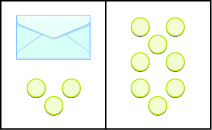What steps are you taking in your mind to figure out how many counters are in the envelope? Perhaps you are thinking “I need to remove the $3$ counters from the left side to get the envelope by itself. Those $3$ counters on the left match with $3$ on the right, so I can take them away from both sides. That leaves five counters on the right, so there must be $5$ counters in the envelope.”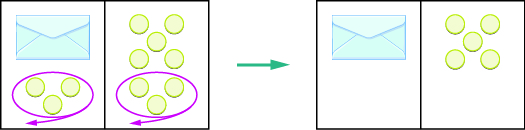What algebraic equation is modeled by this situation? Each side of the desk represents an expression and the center line takes the place of the equal sign. We will call the contents of the envelope $x$, so the number of counters on the left side of the desk is $x+3$. On the right side of the desk are $8$ counters. We are told that $x+3$ is equal to $8$ so our equation is $x+3=8$.$x+3=8$

Let’s write algebraically the steps we took to discover how many counters were in the envelope.

 $x+3=8$ First, we took away three from each side. $x+3\color{red}{-3}=8\color {red}{-3}$ Then we were left with five. $x=5$

Now let’s check our solution. We substitute $5$ for $x$ in the original equation and see if we get a true statement.

$x+3=8$

$\color{red}{5}+3=8$

$8=8\quad\checkmark$  Our solution is correct. Five counters in the envelope plus three more equals eight.

example

Write an equation modeled by the envelopes and counters, and then solve the equation: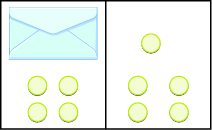Solution

 On the left, write $x$ for the contents of the envelope, add the $4$ counters, so we have $x+4$ . $x+4$ On the right, there are $5$ counters. $5$ The two sides are equal. $x+4=5$ Solve the equation by subtracting $4$ counters from each side.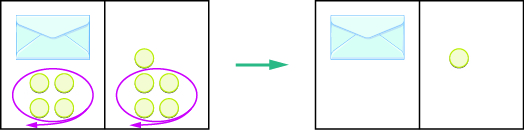We can see that there is one counter in the envelope. This can be shown algebraically as:

$x+4=5$

$x+4\color{red}{-4}=5\color{red}{-4}$

$x=1$

Substitute $1$ for $x$ in the equation to check.

$x+4=5$

$\color{red}{1}+4=5$

$5=5\quad\checkmark$

Since $x=1$ makes the statement true, we know that $1$ is indeed a solution.

try it

Write the equation modeled by the envelopes and counters, and then solve the equation: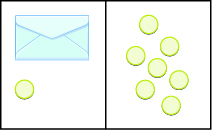Write the equation modeled by the envelopes and counters, and then solve the equation:Solve Equations Using the Subtraction Property of Equality

Our puzzle has given us an idea of what we need to do to solve an equation. The goal is to isolate the variable by itself on one side of the equation. In the previous examples, we used the Subtraction Property of Equality, which states that when we subtract the same quantity from both sides of an equation, we still have equality.

Subtraction Property of Equality

For any numbers $a,b$, and $c$,

if $a=b$

then $a-c=b-c$

Think about twin brothers Andy and Bobby. They are $17$ years old. How old was Andy $3$ years ago? He was $3$ years less than $17$, so his age was $17 - 3$, or $14$. What about Bobby’s age $3$ years ago? Of course, he was $14$ also. Their ages are equal now, and subtracting the same quantity from both of them resulted in equal ages $3$ years ago.

$\begin{array}{c}a=b\\ a - 3=b - 3\end{array}$

Solve an equation using the Subtraction Property of Equality

1. Use the Subtraction Property of Equality to isolate the variable.
2. Simplify the expressions on both sides of the equation.
3. Check the solution.

example

Solve: $x+8=17$.

example

Solve: $100=y+74$.

Solve Equations Using the Addition Property of Equality

In all the equations we have solved so far, a number was added to the variable on one side of the equation. We used subtraction to “undo” the addition in order to isolate the variable.

But suppose we have an equation with a number subtracted from the variable, such as $x - 5=8$. We want to isolate the variable, so to “undo” the subtraction we will add the number to both sides.

We use the Addition Property of Equality, which says we can add the same number to both sides of the equation without changing the equality. Notice how it mirrors the Subtraction Property of Equality.

For any numbers $a,b$ , and $c$ ,

if $a=b$

then $a+c=b+c$

Remember the $17\text{-year-old}$ twins, Andy and Bobby? In ten years, Andy’s age will still equal Bobby’s age. They will both be $27$.

$\begin{array}{c}a=b\\ a+10=b+10\end{array}$
We can add the same number to both sides and still keep the equality.

Solve an equation using the Addition Property of Equality

1. Use the Addition Property of Equality to isolate the variable.
2. Simplify the expressions on both sides of the equation.
3. Check the solution.

example

Solve: $x - 5=8$.

Solution
We will use the Addition Property of Equality to isolate the variable.

 $x-5=8$ Add $5$ to both sides. $x-5\color{red}{+5}=8\color{red}{+5}$ Simplify. $x=13$ Now we can check. Let $x=\color{red}{13}$. $x-5=8$ $\color{red}{13}-5=8$ $8=8\quad\checkmark$

example

Solve: $27=a - 16$.

try it

In the following video we show more examples of how to use the addition and subtraction properties of equality to solve one step linear equations involving whole numbers.